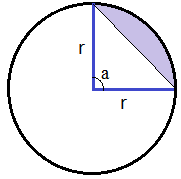## Segment of Circle Calculator

 Radius (r): Arc Angle (a): radian  ̊ degree  π  π/3 Segment Area : Arc Length : Chord Length : Segment perimeter :

A segment is the shape formed between the chord and the arc.

1. Area of shaded part = r2 * (a - sin(a)) / 2

2. arc length of shaded part = r * a

3. Perimeter of segment = r * a + chord length

( Note: unit of arc angle is radian )Thinkcalculator.com provides you helpful and handy calculator resources.